Courses

# Paper Cutting Quiz I, Non Verbal Reasoning

## 25 Questions MCQ Test Non Verbal Reasoning | Paper Cutting Quiz I, Non Verbal Reasoning

Description
This mock test of Paper Cutting Quiz I, Non Verbal Reasoning for LR helps you for every LR entrance exam. This contains 25 Multiple Choice Questions for LR Paper Cutting Quiz I, Non Verbal Reasoning (mcq) to study with solutions a complete question bank. The solved questions answers in this Paper Cutting Quiz I, Non Verbal Reasoning quiz give you a good mix of easy questions and tough questions. LR students definitely take this Paper Cutting Quiz I, Non Verbal Reasoning exercise for a better result in the exam. You can find other Paper Cutting Quiz I, Non Verbal Reasoning extra questions, long questions & short questions for LR on EduRev as well by searching above.
QUESTION: 1

Solution:
QUESTION: 2

Solution:
QUESTION: 3

### Directions to Solve Each of the following questions consists of a set of three figures X, Y and Z showing a sequence of folding of apiece of paper. Figure (Z) shows the manner in which the folded paper has been cut. These three figures are followed by four answer figures from which you have to choose a figure which would most closely resemble the unfolded form of figure (Z). Question - Choose a figure which would most closely resemble the unfolded form of Figure (Z).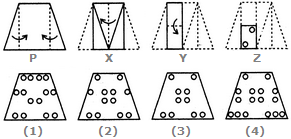Solution:
QUESTION: 4
Directions to Solve

Each of the following questions consists of a set of three figures X, Y and Z showing a sequence of folding of apiece of paper. Figure (Z) shows the manner in which the folded paper has been cut. These three figures are followed by four answer figures from which you have to choose a figure which would most closely resemble the unfolded form of figure (Z).

Question -

Choose a figure which would most closely resemble the unfolded form of Figure (Z).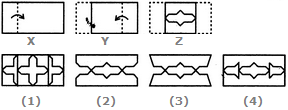Solution:
QUESTION: 5
Directions to Solve

Each of the following questions consists of a set of three figures X, Y and Z showing a sequence of folding of apiece of paper. Figure (Z) shows the manner in which the folded paper has been cut. These three figures are followed by four answer figures from which you have to choose a figure which would most closely resemble the unfolded form of figure (Z).

Question -

Choose a figure which would most closely resemble the unfolded form of Figure (Z).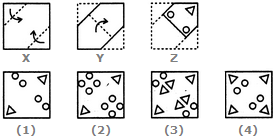Solution:
QUESTION: 6
Directions to Solve

Each of the following questions consists of a set of three figures X, Y and Z showing a sequence of folding of apiece of paper. Figure (Z) shows the manner in which the folded paper has been cut. These three figures are followed by four answer figures from which you have to choose a figure which would most closely resemble the unfolded form of figure (Z).

Question -

Choose a figure which would most closely resemble the unfolded form of Figure (Z).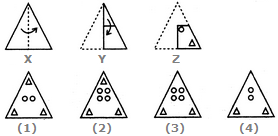Solution:
QUESTION: 7
Directions to Solve

Each of the following questions consists of a set of three figures X, Y and Z showing a sequence of folding of apiece of paper. Figure (Z) shows the manner in which the folded paper has been cut. These three figures are followed by four answer figures from which you have to choose a figure which would most closely resemble the unfolded form of figure (Z).

Question -

Choose a figure which would most closely resemble the unfolded form of Figure (Z).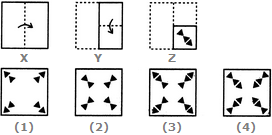Solution:
QUESTION: 8
Directions to Solve

Each of the following questions consists of a set of three figures X, Y and Z showing a sequence of folding of apiece of paper. Figure (Z) shows the manner in which the folded paper has been cut. These three figures are followed by four answer figures from which you have to choose a figure which would most closely resemble the unfolded form of figure (Z).

Question -

Choose a figure which would most closely resemble the unfolded form of Figure (Z).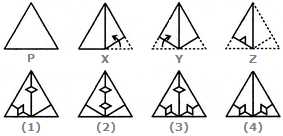Solution:
QUESTION: 9
Directions to Solve

Each of the following questions consists of a set of three figures X, Y and Z showing a sequence of folding of apiece of paper. Figure (Z) shows the manner in which the folded paper has been cut. These three figures are followed by four answer figures from which you have to choose a figure which would most closely resemble the unfolded form of figure (Z).

Question -

Choose a figure which would most closely resemble the unfolded form of Figure (Z).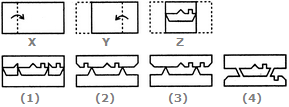Solution:
QUESTION: 10
Directions to Solve

Each of the following questions consists of a set of three figures X, Y and Z showing a sequence of folding of apiece of paper. Figure (Z) shows the manner in which the folded paper has been cut. These three figures are followed by four answer figures from which you have to choose a figure which would most closely resemble the unfolded form of figure (Z).

Question -

Choose a figure which would most closely resemble the unfolded form of Figure (Z).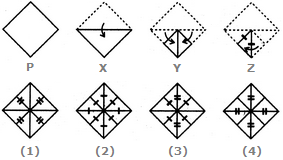Solution:
QUESTION: 11
Directions to Solve

Each of the following questions consists of a set of three figures X, Y and Z showing a sequence of folding of apiece of paper. Figure (Z) shows the manner in which the folded paper has been cut. These three figures are followed by four answer figures from which you have to choose a figure which would most closely resemble the unfolded form of figure (Z).

Question -

Choose a figure which would most closely resemble the unfolded form of Figure (Z).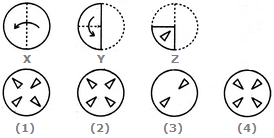Solution:
QUESTION: 12
Directions to Solve

Each of the following questions consists of a set of three figures X, Y and Z showing a sequence of folding of apiece of paper. Figure (Z) shows the manner in which the folded paper has been cut. These three figures are followed by four answer figures from which you have to choose a figure which would most closely resemble the unfolded form of figure (Z).

Question -

Choose a figure which would most closely resemble the unfolded form of Figure (Z).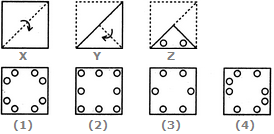Solution:
QUESTION: 13
Directions to Solve

Each of the following questions consists of a set of three figures X, Y and Z showing a sequence of folding of apiece of paper. Figure (Z) shows the manner in which the folded paper has been cut. These three figures are followed by four answer figures from which you have to choose a figure which would most closely resemble the unfolded form of figure (Z).

Question -

Choose a figure which would most closely resemble the unfolded form of Figure (Z).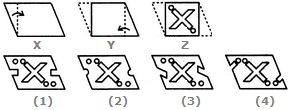Solution:
QUESTION: 14
Directions to Solve

Each of the following questions consists of a set of three figures X, Y and Z showing a sequence of folding of apiece of paper. Figure (Z) shows the manner in which the folded paper has been cut. These three figures are followed by four answer figures from which you have to choose a figure which would most closely resemble the unfolded form of figure (Z).

Question -

Choose a figure which would most closely resemble the unfolded form of Figure (Z).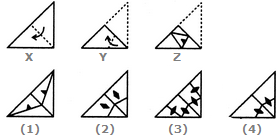Solution:
QUESTION: 15
Directions to Solve

Each of the following questions consists of a set of three figures X, Y and Z showing a sequence of folding of apiece of paper. Figure (Z) shows the manner in which the folded paper has been cut. These three figures are followed by four answer figures from which you have to choose a figure which would most closely resemble the unfolded form of figure (Z).

Question -

Choose a figure which would most closely resemble the unfolded form of Figure (Z).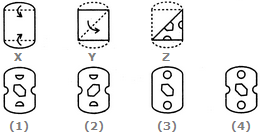Solution:
QUESTION: 16
Directions to Solve

Each of the following questions consists of a set of three figures X, Y and Z showing a sequence of folding of apiece of paper. Figure (Z) shows the manner in which the folded paper has been cut. These three figures are followed by four answer figures from which you have to choose a figure which would most closely resemble the unfolded form of figure (Z).

Question -

Choose a figure which would most closely resemble the unfolded form of Figure (Z).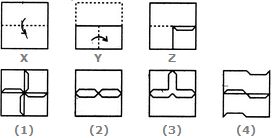Solution:
QUESTION: 17
Directions to Solve

Each of the following questions consists of a set of three figures X, Y and Z showing a sequence of folding of apiece of paper. Figure (Z) shows the manner in which the folded paper has been cut. These three figures are followed by four answer figures from which you have to choose a figure which would most closely resemble the unfolded form of figure (Z).

Question -

Choose a figure which would most closely resemble the unfolded form of Figure (Z).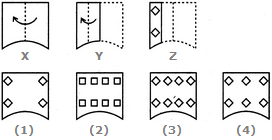Solution:
QUESTION: 18
Directions to Solve

Each of the following questions consists of a set of three figures X, Y and Z showing a sequence of folding of apiece of paper. Figure (Z) shows the manner in which the folded paper has been cut. These three figures are followed by four answer figures from which you have to choose a figure which would most closely resemble the unfolded form of figure (Z).

Question -

Choose a figure which would most closely resemble the unfolded form of Figure (Z).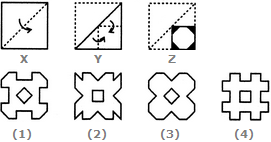Solution:
QUESTION: 19
Directions to Solve

Each of the following questions consists of a set of three figures X, Y and Z showing a sequence of folding of apiece of paper. Figure (Z) shows the manner in which the folded paper has been cut. These three figures are followed by four answer figures from which you have to choose a figure which would most closely resemble the unfolded form of figure (Z).

Question -

Choose a figure which would most closely resemble the unfolded form of Figure (Z).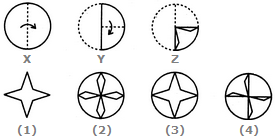Solution:
QUESTION: 20
Directions to Solve

Each of the following questions consists of a set of three figures X, Y and Z showing a sequence of folding of apiece of paper. Figure (Z) shows the manner in which the folded paper has been cut. These three figures are followed by four answer figures from which you have to choose a figure which would most closely resemble the unfolded form of figure (Z).

Question -

Choose a figure which would most closely resemble the unfolded form of Figure (Z).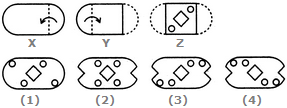Solution:
QUESTION: 21
Directions to Solve

Each of the following questions consists of a set of three figures X, Y and Z showing a sequence of folding of apiece of paper. Figure (Z) shows the manner in which the folded paper has been cut. These three figures are followed by four answer figures from which you have to choose a figure which would most closely resemble the unfolded form of figure (Z).

Question -

Choose a figure which would most closely resemble the unfolded form of Figure (Z).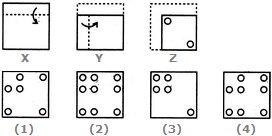Solution:
QUESTION: 22
Directions to Solve

Each of the following questions consists of a set of three figures X, Y and Z showing a sequence of folding of apiece of paper. Figure (Z) shows the manner in which the folded paper has been cut. These three figures are followed by four answer figures from which you have to choose a figure which would most closely resemble the unfolded form of figure (Z).

Question -

Choose a figure which would most closely resemble the unfolded form of Figure (Z).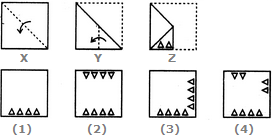Solution:
QUESTION: 23
Directions to Solve

Each of the following questions consists of a set of three figures X, Y and Z showing a sequence of folding of apiece of paper. Figure (Z) shows the manner in which the folded paper has been cut. These three figures are followed by four answer figures from which you have to choose a figure which would most closely resemble the unfolded form of figure (Z).

Question -

Choose a figure which would most closely resemble the unfolded form of Figure (Z).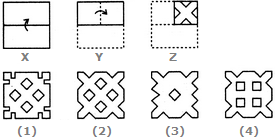Solution:
QUESTION: 24
Directions to Solve

Each of the following questions consists of a set of three figures X, Y and Z showing a sequence of folding of apiece of paper. Figure (Z) shows the manner in which the folded paper has been cut. These three figures are followed by four answer figures from which you have to choose a figure which would most closely resemble the unfolded form of figure (Z).

Question -

Choose a figure which would most closely resemble the unfolded form of Figure (Z).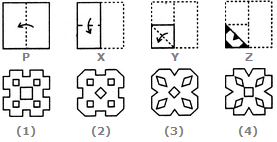Solution:
QUESTION: 25
Directions to Solve

Each of the following questions consists of a set of three figures X, Y and Z showing a sequence of folding of apiece of paper. Figure (Z) shows the manner in which the folded paper has been cut. These three figures are followed by four answer figures from which you have to choose a figure which would most closely resemble the unfolded form of figure (Z).

Question -

Choose a figure which would most closely resemble the unfolded form of Figure (Z).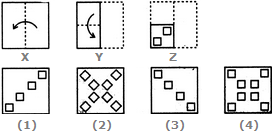Solution: